# 4 Advanced Control Flow Written by Matt Galloway

In the previous chapter, you learned how to control the flow of execution using the decision-making powers of `if` statements and the `while` loop. In this chapter, you’ll continue to learn how to control the flow of execution. You’ll learn about another loop known as the `for` loop.

Loops may not sound very interesting, but they’re very common in computer programs. For example, you might have code to download an image from the cloud; with a loop, you could run that multiple times to download your entire photo library. Or, if you have a game with multiple computer-controlled characters, you might need a loop to go through each one and make sure it knows what to do next.

You’ll also learn about `switch` statements, which are particularly powerful in Swift. They let you inspect a value and decide what to do based on that value. They’re incredibly powerful when used with some advanced Swift features such as pattern matching.

## Countable ranges

Before you dive into the `for` loop statement, you need to know about the Countable Range data types, which let you represent a sequence of countable integers. Let’s look at two types of ranges.

First, there’s countable closed range, which you represent like so:

``````let closedRange = 0...5
``````

The three dots (`...`) indicate that this range is closed, which means the range goes from 0 to 5 inclusive. That’s the numbers `(0, 1, 2, 3, 4, 5)`.

Second, there’s countable half-open range, which you represent like so:

``````let halfOpenRange = 0..<5
``````

Here, you replace the three dots with two dots and a less-than sign (`..<`). Half-open means the range goes from 0 up to, but not including, 5. That’s the numbers `(0, 1, 2, 3, 4)`.

Both open and half-open ranges must always be increasing. In other words, the second number must always be greater than or equal to the first. Countable ranges are commonly used in both `for` loops and `switch` statements, which means that throughout the rest of the chapter, you’ll use ranges as well!

### A random interlude

A common need in programming is to be able to generate random numbers. And Swift provides the functionality built into the language, which is pretty handy!

As an example, imagine an application that needs to simulate rolling a die. You may want to do something until the code rolls a six. Now that you know about `while` loops, you can do that with the `random` feature. You could do that like so:

``````while Int.random(in: 1...6) != 6 {
print("Not a six")
}
``````

Note: The `random(in:)` here is a call to a function. You’ll see more about these in Chapter 5, “Functions”, and how they apply to types in Chapter 12, “Methods”.

## For loops

In the previous chapter you looked at `while` loops. Now that you know about ranges, it’s time to look at another type of loop: the `for` loop. This is probably the most common loop you’ll see, and you’ll use it to run code a certain number of times.

You construct a `for` loop like this:

``````for <CONSTANT> in <COUNTABLE RANGE> {
<LOOP CODE>
}
``````

The loop begins with the `for` keyword, followed by a name given to the loop constant (more on that shortly), followed by `in`, followed by the range to loop through. Here’s an example:

``````let count = 10
var sum = 0
for i in 1...count {
sum += i
}
``````

In the code above, the `for` loop iterates through the range 1 to `count`. At the first iteration, `i` will equal the first element in the range: 1. Each time around the loop, `i` will increment until it’s equal to `count`; the loop will execute one final time and then finish.

Note: If you’d used a half-open range, the last iteration would see `i` equal to `count - 1`.

Inside the loop, you add `i` to the `sum` variable; it runs 10 times to calculate the sequence `1 + 2 + 3 + 4 + 5 + ...` all the way up to 10.

Here are the values of the constant `i` and variable `sum` for each iteration:

• Start of iteration 1: `i` = 1, `sum` = 0
• Start of iteration 2: `i` = 2, `sum` = 1
• Start of iteration 3: `i` = 3, `sum` = 3
• Start of iteration 4: `i` = 4, `sum` = 6
• Start of iteration 5: `i` = 5, `sum` = 10
• Start of iteration 6: `i` = 6, `sum` = 15
• Start of iteration 7: `i` = 7, `sum` = 21
• Start of iteration 8: `i` = 8, `sum` = 28
• Start of iteration 9: `i` = 9, `sum` = 36
• Start of iteration 10: `i` = 10, `sum` = 45
• After iteration 10: `sum` = 55

In terms of scope, the `i` constant is only visible inside the scope of the `for` loop, which means it’s not available outside of the loop.

Note: If you’re mathematically astute, you might notice that this example computes triangle numbers. Here’s a quick explanation: http://bbc.in/1O89TGP

Xcode’s playground gives you a handy way to visualize such an iteration. Hover over the `sum += i` line in the results pane, and you’ll see a white dot on the right. Hover over that dot to reveal a plus (+) button: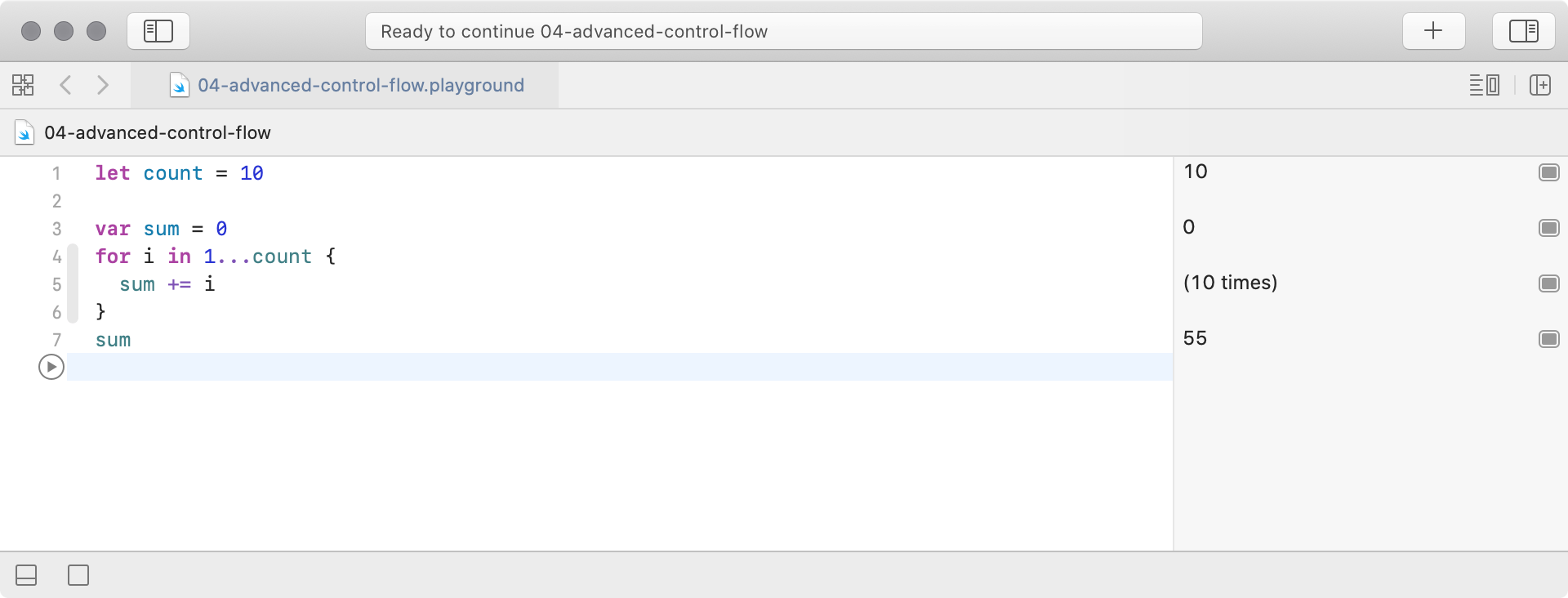Click this plus (+) button and Xcode will display a graph underneath the line within the playground code editor: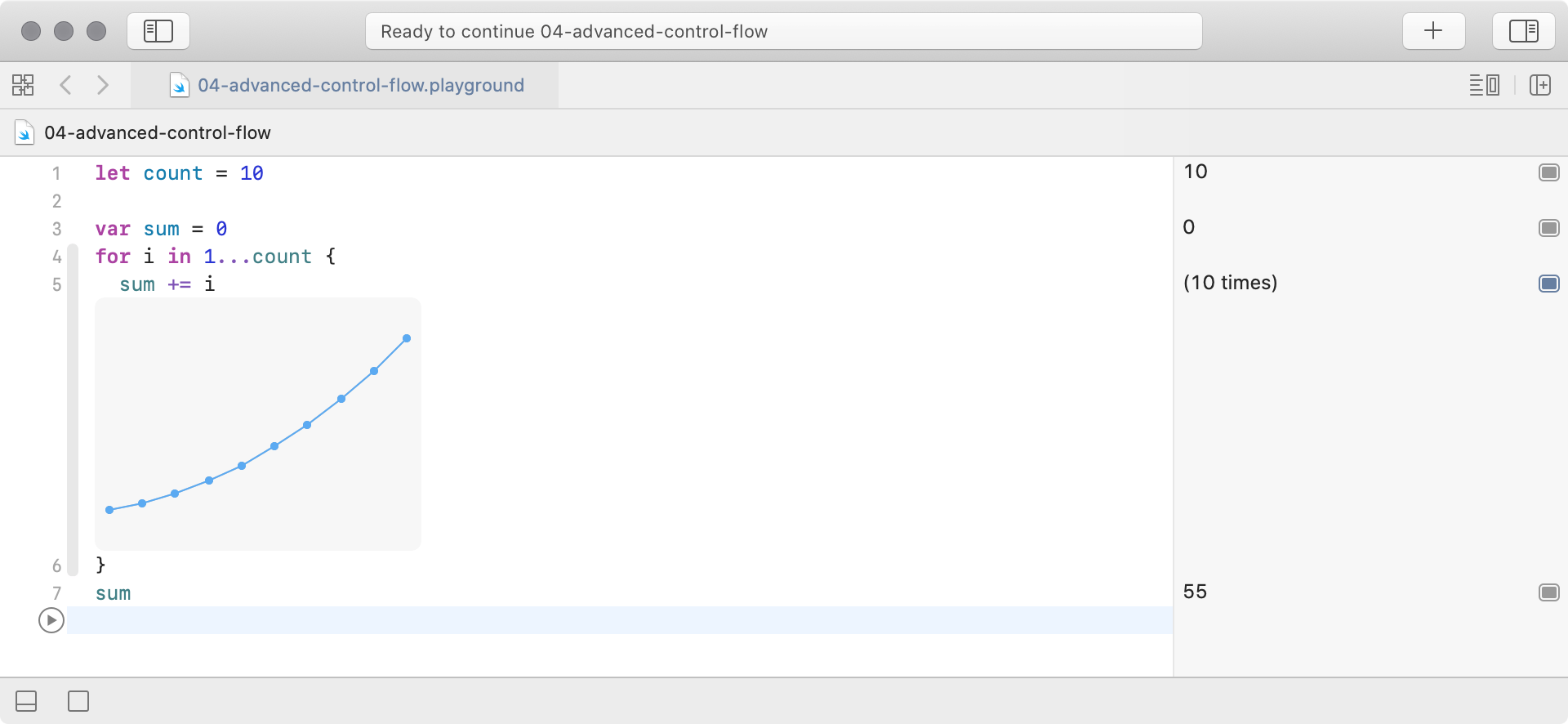This graph lets you visualize the `sum` variable as the loop iterates.

Finally, sometimes you only want to loop a certain number of times, so you don’t need to use the loop constant at all.

In that case, you can employ the underscore to indicate you’re ignoring it, like so:

``````sum = 1
var lastSum = 0

for _ in 0..<count {
let temp = sum
sum = sum + lastSum
lastSum = temp
}
``````

This code doesn’t require a loop constant; the loop simply needs to run a certain number of times. In this case, the range is 0 up to, but not including, `count` and is half-open. This is the usual way of writing loops that run a certain number of times. It’s also possible to only perform the iteration under certain conditions. For example, imagine you wanted to compute a sum similar to that of triangle numbers, but only for odd numbers:

``````sum = 0
for i in 1...count where i % 2 == 1 {
sum += i
}
``````

The previous loop has a `where` clause in the `for` loop statement. The loop still runs through all values in the range 1 to `count`, but it will only execute the loop’s code block when the `where` condition is true; in this case, where `i` is odd.

### Continue and labeled statements

Sometimes you’d like to skip a loop iteration for a particular case without breaking out of the loop entirely. You can do this with the `continue` statement, which immediately ends the current iteration of the loop and starts the next iteration.

Note: In many cases, you can use the simpler `where` clause you just learned about. The `continue` statement gives you a higher level of control, letting you decide where and when you want to skip an iteration.

Take the example of an eight by eight grid, where each cell holds a value of the row multiplied by the column. It looks much like a multiplication table, doesn’t it?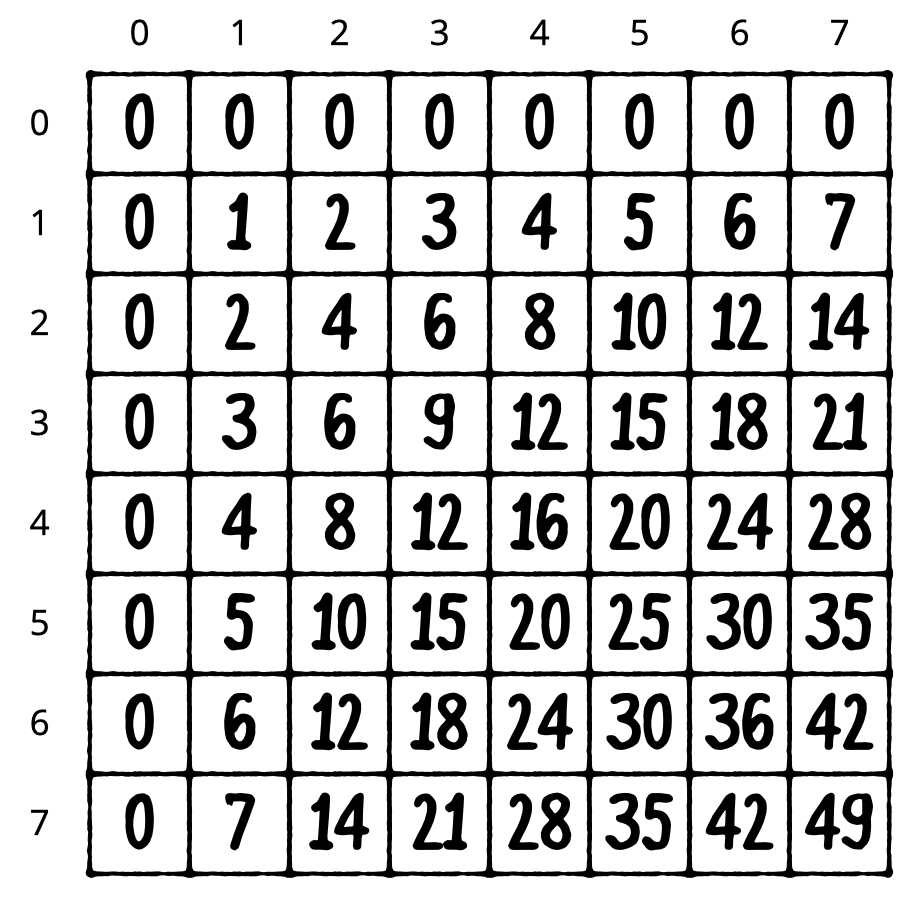Let’s say you wanted to calculate the sum of all cells but exclude all even rows, as shown below: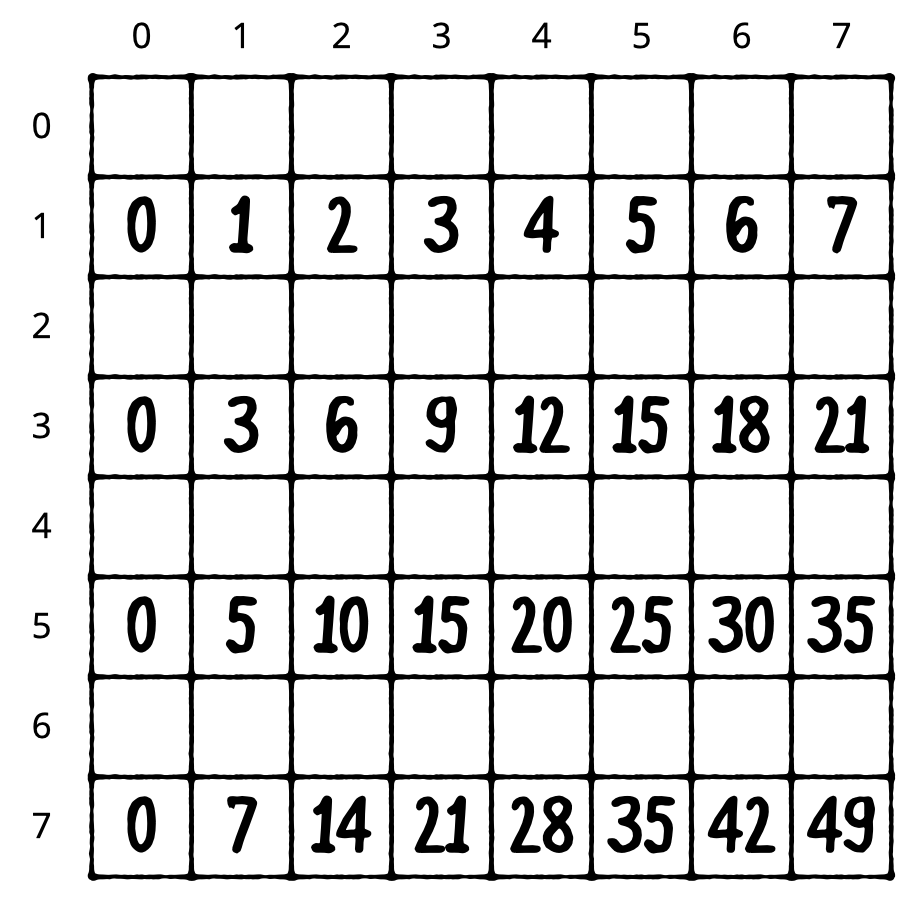Using a `for` loop, you can achieve this as follows:

``````sum = 0

for row in 0..<8 {
if row % 2 == 0 {
continue
}

for column in 0..<8 {
sum += row * column
}
}
``````

When the row modulo 2 equals 0, the row is even. In this case, `continue` makes the `for` loop skip to the next row. Just like `break`, `continue` works with both `for` loops and `while` loops.

The second code example will calculate the sum of all cells, excluding those where the column is greater than or equal to the row.

To illustrate, it should sum the following cells: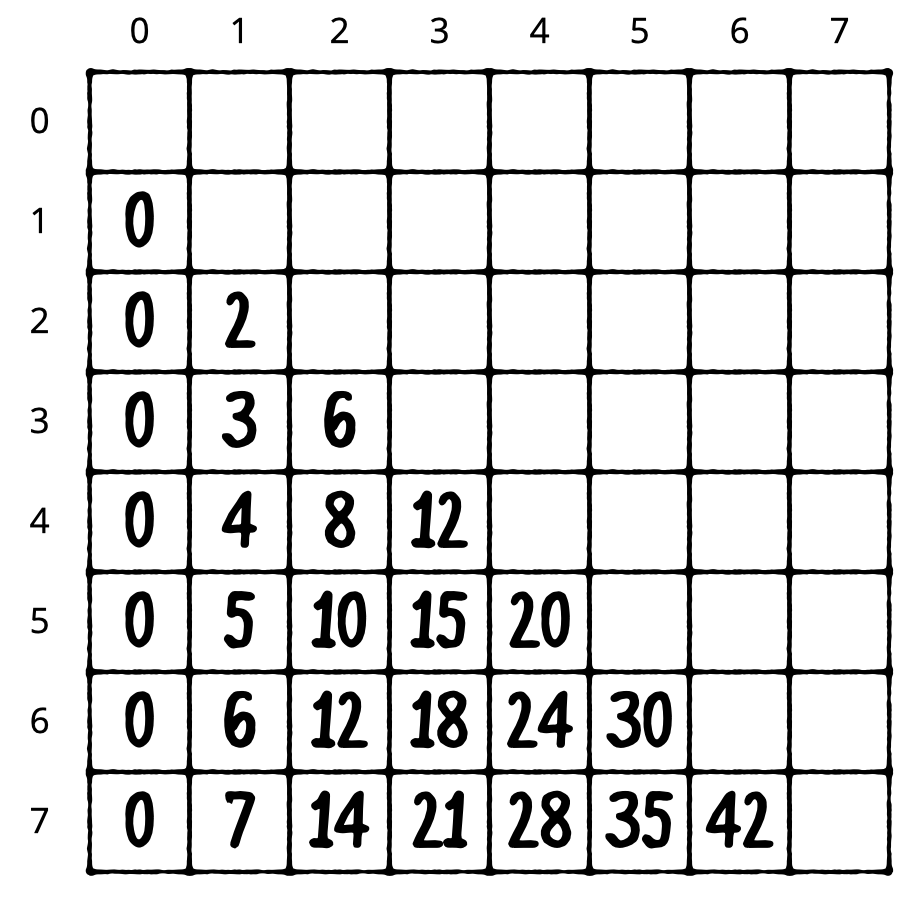Using a `for` loop, you can achieve this as follows:

``````sum = 0

rowLoop: for row in 0..<8 {
columnLoop: for column in 0..<8 {
if row == column {
continue rowLoop
}
sum += row * column
}
}
``````

The previous code block makes use of labeled statements, labeling the two loops as `rowLoop` and the `columnLoop`, respectively. When the row equals the column inside the inner `columnLoop`, the outer `rowLoop` will continue.

You can use labeled statements like these with `break` to break out of a certain loop. Normally, `break` and `continue` work on the innermost loop, so you need to use labeled statements to act on the outer loop.

### Mini-exercises

1. Create a constant named `range`, and set it equal to a range starting at 1 and ending with 10 inclusive. Write a `for` loop that iterates over this range and prints the square of each number.

2. Write a `for` loop to iterate over the same range as in the exercise above and print the square root of each number. You’ll need to type convert your loop constant.

3. Above, you saw a `for` loop that iterated over only the even rows like so:

``````sum = 0
for row in 0..<8 {
if row % 2 == 0 {
continue
}
for column in 0..<8 {
sum += row * column
}
}
``````

Change this to use a `where` clause on the first `for` loop to skip even rows instead of using `continue`. Check that the sum is 448, as in the initial example.

## Switch statements

You can also control flow via the `switch` statement. It executes different code depending on the value of a variable or constant. Here’s a `switch` statement that acts on an integer:

``````let number = 10

switch number {
case 0:
print("Zero")
default:
print("Non-zero")
}
``````

In this example, the code will print the following:

`Non-zero`

The purpose of this `switch` statement is to determine whether or not a number is zero. It will get more complex — I promise!

To handle a specific case, you use `case` followed by the value you want to check for, which in this case is `0`. Then, you use `default` to signify what should happen for all other values.

Here’s another example:

``````switch number {
case 10:
print("It’s ten!")
default:
break
}
``````

This time you check for 10, in which case, you print a message. Nothing should happen for other values. When you want nothing to happen for a case, you use the `break` statement. This tells Swift that you meant to not write any code here and that nothing should happen. Cases can never be empty, so you must write some code, even if it’s just a `break`!

Of course, `switch` statements also work with data types other than integers. They work with any data type!

Here’s an example of switching on a string:

``````let string = "Dog"

switch string {
case "Cat", "Dog":
print("Animal is a house pet.")
default:
print("Animal is not a house pet.")
}
``````

This will print the following:

`Animal is a house pet.`

In this example, you provide two values for the case, meaning that if the value is equal to either `"Cat"` or `"Dog"`, then the statement will execute the case.

### Advanced switch statements

You can also give your `switch` statements more than one case. In the previous chapter, you saw an `if` statement that used multiple `else` clauses to convert an hour of the day to a string describing that part of the day. You could rewrite that more succinctly with a `switch` statement, like so:

``````let hourOfDay = 12
var timeOfDay = ""

switch hourOfDay {
case 0, 1, 2, 3, 4, 5:
timeOfDay = "Early morning"
case 6, 7, 8, 9, 10, 11:
timeOfDay = "Morning"
case 12, 13, 14, 15, 16:
timeOfDay = "Afternoon"
case 17, 18, 19:
timeOfDay = "Evening"
case 20, 21, 22, 23:
timeOfDay = "Late evening"
default:
timeOfDay = "INVALID HOUR!"
}

print(timeOfDay)
``````

This code will print the following:

`Afternoon`

Remember ranges? Well, you can use ranges to simplify this `switch` statement. You can rewrite the above code using ranges:

``````switch hourOfDay {
case 0...5:
timeOfDay = "Early morning"
case 6...11:
timeOfDay = "Morning"
case 12...16:
timeOfDay = "Afternoon"
case 17...19:
timeOfDay = "Evening"
case 20..<24:
timeOfDay = "Late evening"
default:
timeOfDay = "INVALID HOUR!"
}
``````

This code is more concise than writing out each value individually for all cases.

When there are multiple cases, the statement will execute the first one that matches. You’ll probably agree that this is more concise and clear than using an `if` statement for this example.

It’s slightly more precise as well because the `if` statement method didn’t address negative numbers, which here are correctly deemed to be invalid.

It’s also possible to match a case to a condition based on a property of the value. As you learned in Chapter 2, “Types & Operations” you can use the modulo operator to determine if an integer is even or odd.

Consider this code:

``````switch number {
case let x where x % 2 == 0:
print("Even")
default:
print("Odd")
}
``````

This will print the following:

`Even`

This `switch` statement uses the `let-where` syntax, meaning the case will match only when a certain condition is true. The `let` part binds a value to a name, while the `where` part provides a Boolean condition that must be true for the case to match.

In this example, you’ve designed the case to match if the value is even — that is, if the value modulo 2 equals 0.

The method by which you can match values based on conditions is known as pattern matching.

In the previous example, the binding introduced an unnecessary constant `x`; it’s merely another name for `number`.

You are allowed to use `number` in the `where` clause, and replace the binding with an underscore to ignore it.

``````switch number {
case _ where number % 2 == 0:
print("Even")
default:
print("Odd")
}
``````

### Partial matching

Another way you can use `switch` statements with matching to great effect is as follows:

``````let coordinates = (x: 3, y: 2, z: 5)

switch coordinates {
case (0, 0, 0): // 1
print("Origin")
case (_, 0, 0): // 2
print("On the x-axis.")
case (0, _, 0): // 3
print("On the y-axis.")
case (0, 0, _): // 4
print("On the z-axis.")
default:        // 5
print("Somewhere in space")
}
``````

This `switch` statement makes use of partial matching. Here’s what each case does, in order:

1. Matches precisely the case where the value is `(0, 0, 0)`. This is the origin of 3D space.

2. Matches y=0, z=0 and any value of x. This means the coordinate is on the x-axis.

3. Matches x=0, z=0 and any value of y. This means the coordinate is on the y-axis.

4. Matches x=0, y=0 and any value of z. This means the coordinate is on the z-axis.

5. Matches the remainder of coordinates.

You’re using the underscore to mean that you don’t care about the value. If you don’t want to ignore the value, you can bind it and use it in your `switch` statement.

Here’s an example of how to do this:

``````switch coordinates {
case (0, 0, 0):
print("Origin")
case (let x, 0, 0):
print("On the x-axis at x = \(x)")
case (0, let y, 0):
print("On the y-axis at y = \(y)")
case (0, 0, let z):
print("On the z-axis at z = \(z)")
case let (x, y, z):
print("Somewhere in space at x = \(x), y = \(y), z = \(z)")
}
``````

Here, the axis cases use the `let` syntax to pull out the pertinent values. The code then prints the values using string interpolation to build the string.

Notice how you don’t need a default in this `switch` statement. This is because the final case is essentially the default; it matches anything because there are no constraints on any part of the tuple. If the `switch` statement exhausts all possible values with its cases, no default is necessary.

Also notice how you could use a single `let` to bind all values of the tuple: `let (x, y, z)` is the same as `(let x, let y, let z)`.

Finally, you can use the same `let-where` syntax you saw earlier to match more complex cases. For example:

``````switch coordinates {
case let (x, y, _) where y == x:
print("Along the y = x line.")
case let (x, y, _) where y == x * x:
print("Along the y = x^2 line.")
default:
break
}
``````

Here, you match the “y equals x” and “y equals x squared” lines.

And those are the basics of `switch` statements!

### Mini-exercises

1. Write a switch statement that takes an age as an integer and prints out the life stage related to that age. You can make up the life stages, or use my categorization as follows: 0-2 years, Infant; 3-12 years, Child; 13-19 years, Teenager; 20-39, Adult; 40-60, Middle-aged; 61+, Elderly.

2. Write a `switch` statement that takes a tuple containing a string and an integer. The string is a name, and the integer is an age. Use the same cases you used in the previous exercise and `let` syntax to print out the name followed by the life stage. For example, for myself, it would print out `"Matt is an adult."`.

## Challenges

Before moving on, here are some challenges to test your knowledge of advanced control flow. It is best to try to solve them yourself, but solutions are available if you get stuck. These came with the download or are available at the printed book’s source code link listed in the introduction.

### Challenge 1: How many times

In the following `for` loop, what will be the value of `sum`, and how many iterations will happen?

``````var sum = 0
for i in 0...5 {
sum += i
}
``````

### Challenge 2: Count the letter

In the `while` loop below, how many instances of “a” will there be in `aLotOfAs`? Hint: `aLotOfAs.count` tells you how many characters are in the string `aLotOfAs`.

``````var aLotOfAs = ""
while aLotOfAs.count < 10 {
aLotOfAs += "a"
}
``````

### Challenge 3: What will print

Consider the following `switch` statement:

``````switch coordinates {
case let (x, y, z) where x == y && y == z:
print("x = y = z")
case (_, _, 0):
print("On the x/y plane")
case (_, 0, _):
print("On the x/z plane")
case (0, _, _):
print("On the y/z plane")
default:
print("Nothing special")
}
``````

What will this code print when `coordinates` is each of the following?

``````let coordinates = (1, 5, 0)
let coordinates = (2, 2, 2)
let coordinates = (3, 0, 1)
let coordinates = (3, 2, 5)
let coordinates = (0, 2, 4)
``````

### Challenge 4: Closed range size

A closed range can never be empty. Why?

### Challenge 5: The final countdown

Print a countdown from 10 to 0. (Note: do not use the `reversed()` method, which will be introduced later.)

### Challenge 6: Print a sequence

Print 0.0, 0.1, 0.2, 0.3, 0.4, 0.5, 0.6, 0.7, 0.8, 0.9, 1.0. (Note: do not use the `stride(from:by:to:)` function, which will be introduced later.)

## Key points

• You can use countable ranges to create a sequence of integers, incrementing to move from one value to another.
• Closed ranges include both the start and end values.
• Half-open ranges include the start value and stop one before the end value.
• For loops allow you to iterate over a range.
• The continue statement lets you finish the current iteration of a loop and begin the next iteration.
• Labeled statements let you use `break` and `continue` on an outer loop.
• You use switch statements to decide which code to run depending on the value of a variable or constant.
• The power of a `switch` statement comes from leveraging pattern matching to compare values using complex rules.

Have a technical question? Want to report a bug? You can ask questions and report bugs to the book authors in our official book forum here.

Have feedback to share about the online reading experience? If you have feedback about the UI, UX, highlighting, or other features of our online readers, you can send them to the design team with the form below:

© 2021 Razeware LLC